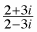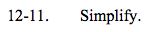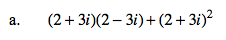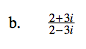### Home > A2C > Chapter 12 > Lesson 12.1.1 > Problem12-11

12-11.
1. Simplify. Homework Help ✎

1. (2 + 3i)(2 − 3i) + (2 + 3i)2

2.4 − (−9) + 4 + 12i + (−9)

8 + 12i$\frac{2+3i}{2-3i}\cdot\frac{2+3i}{2+3i}$

$-\frac{5}{13}+\frac{12}{13}i$